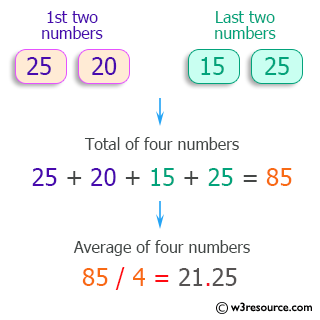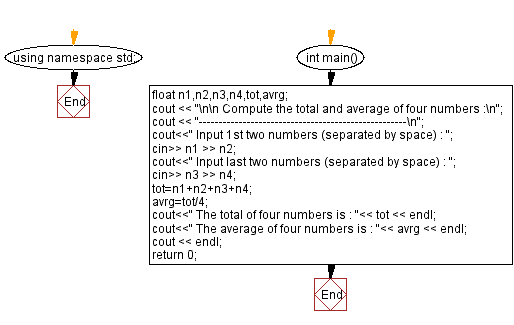﻿ C++ Exercises: Compute the total and average of four numbers - w3resource

# C++ Exercises: Compute the total and average of four numbers

## C++ Basic: Exercise-30 with Solution

Write a C++ program to compute the total and average of four numbers.

Pictorial Presentation:Sample Solution:

C++ Code :

``````#include <iostream>
using namespace std;

int main()
{
float n1,n2,n3,n4,tot,avrg;
cout << "\n\n Compute the total and average of four numbers :\n";
cout << "----------------------------------------------------\n";
cout<<" Input 1st two numbers (separated by space) : ";
cin>> n1 >> n2;
cout<<" Input last two numbers (separated by space) : ";
cin>> n3 >> n4;
tot=n1+n2+n3+n4;
avrg=tot/4;
cout<<" The total of four numbers is : "<< tot << endl;
cout<<" The average of four numbers is : "<< avrg << endl;
cout << endl;
return 0;
}
``````

Sample Output:

``` Compute the total and average of four numbers :
----------------------------------------------------
Input 1st two numbers (separated by space) : 25 20
Input last two numbers (separated by space) : 15 25
The total of four numbers is : 85
The average of four numbers is : 21.25
```

Flowchart:C++ Code Editor:

What is the difficulty level of this exercise?

﻿

## C++ Programming: Tips of the Day

Why is there no std::stou?

The most pat answer would be that the C library has no corresponding "strtou", and the C++11 string functions are all just thinly veiled wrappers around the C library functions: The std::sto* functions mirror strto*, and the std::to_string functions use sprintf.

Ref: https://bit.ly/3wtz2qA

We are closing our Disqus commenting system for some maintenanace issues. You may write to us at reach[at]yahoo[dot]com or visit us at Facebook